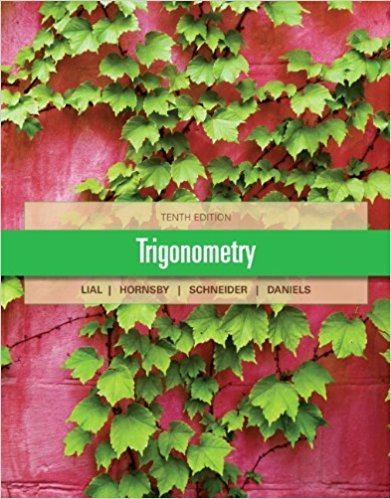×
×

# Solutions for Chapter 2.4: Solving Right Triangles## Full solutions for Trigonometry | 10th Edition

ISBN: 9780321671776Solutions for Chapter 2.4: Solving Right Triangles

Solutions for Chapter 2.4
4 5 0 280 Reviews
25
1
##### ISBN: 9780321671776

This expansive textbook survival guide covers the following chapters and their solutions. Since 58 problems in chapter 2.4: Solving Right Triangles have been answered, more than 34244 students have viewed full step-by-step solutions from this chapter. This textbook survival guide was created for the textbook: Trigonometry, edition: 10. Chapter 2.4: Solving Right Triangles includes 58 full step-by-step solutions. Trigonometry was written by and is associated to the ISBN: 9780321671776.

Key Math Terms and definitions covered in this textbook
• Basis for V.

Independent vectors VI, ... , v d whose linear combinations give each vector in V as v = CIVI + ... + CdVd. V has many bases, each basis gives unique c's. A vector space has many bases!

• Cayley-Hamilton Theorem.

peA) = det(A - AI) has peA) = zero matrix.

• Complete solution x = x p + Xn to Ax = b.

(Particular x p) + (x n in nullspace).

• Free columns of A.

Columns without pivots; these are combinations of earlier columns.

• Free variable Xi.

Column i has no pivot in elimination. We can give the n - r free variables any values, then Ax = b determines the r pivot variables (if solvable!).

• Fundamental Theorem.

The nullspace N (A) and row space C (AT) are orthogonal complements in Rn(perpendicular from Ax = 0 with dimensions rand n - r). Applied to AT, the column space C(A) is the orthogonal complement of N(AT) in Rm.

• Inverse matrix A-I.

Square matrix with A-I A = I and AA-l = I. No inverse if det A = 0 and rank(A) < n and Ax = 0 for a nonzero vector x. The inverses of AB and AT are B-1 A-I and (A-I)T. Cofactor formula (A-l)ij = Cji! detA.

• Kronecker product (tensor product) A ® B.

Blocks aij B, eigenvalues Ap(A)Aq(B).

• Least squares solution X.

The vector x that minimizes the error lie 112 solves AT Ax = ATb. Then e = b - Ax is orthogonal to all columns of A.

• Left nullspace N (AT).

Nullspace of AT = "left nullspace" of A because y T A = OT.

• Minimal polynomial of A.

The lowest degree polynomial with meA) = zero matrix. This is peA) = det(A - AI) if no eigenvalues are repeated; always meA) divides peA).

• Nilpotent matrix N.

Some power of N is the zero matrix, N k = o. The only eigenvalue is A = 0 (repeated n times). Examples: triangular matrices with zero diagonal.

• Particular solution x p.

Any solution to Ax = b; often x p has free variables = o.

• Polar decomposition A = Q H.

Orthogonal Q times positive (semi)definite H.

• Rank r (A)

= number of pivots = dimension of column space = dimension of row space.

• Rayleigh quotient q (x) = X T Ax I x T x for symmetric A: Amin < q (x) < Amax.

Those extremes are reached at the eigenvectors x for Amin(A) and Amax(A).

• Saddle point of I(x}, ... ,xn ).

A point where the first derivatives of I are zero and the second derivative matrix (a2 II aXi ax j = Hessian matrix) is indefinite.

• Singular matrix A.

A square matrix that has no inverse: det(A) = o.

• Trace of A

= sum of diagonal entries = sum of eigenvalues of A. Tr AB = Tr BA.

• Wavelets Wjk(t).

Stretch and shift the time axis to create Wjk(t) = woo(2j t - k).

×